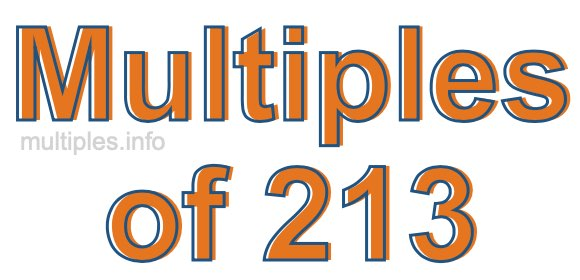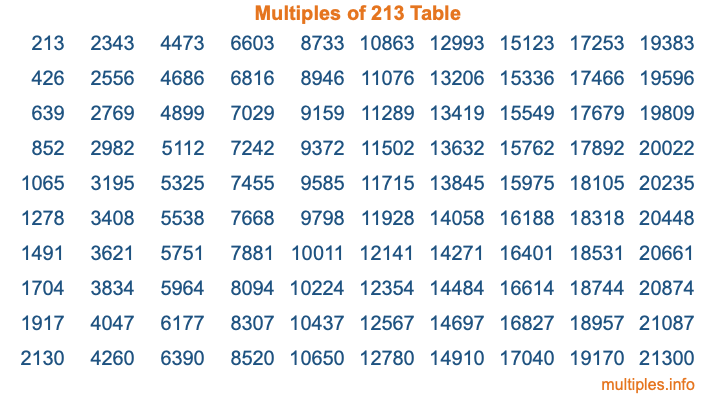Multiples of 213Welcome to the Multiples of 213 page. Here we will first teach you everything you will ever need to know about the multiples of 213, and then give you a study guide summary of everything we taught you to make sure you remember it all. Use this page to look up facts and learn information about the multiples of 213. This page will make you a multiples of two hundred thirteen expert!

Definition of Multiples of 213
Multiples of 213 are all the numbers that when divided by 213 equal an integer. Each of the multiples of 213 are called a multiple. A multiple of 213 is created by multiplying 213 by an integer.

Therefore, to create a list of multiples of 213, you start with 1 multiplied by 213, then 2 multiplied by 213, then 3 multiplied by 213, and so on for as long as you want. Thus, the list of the first five multiples of 213 is 213, 426, 639, 852, and 1065. To see a larger list of multiples of 213, see the printable image of Multiples of 213 further down on this page. We also have a category where you can choose any nth multiple of 213.

Multiples of 213 Checker
The Multiples of 213 Checker below checks to see if any number of your choice is a multiple of 213. In other words, it checks to see if there is any number (integer) that when multiplied by 213 will equal your number. To do that, we divide your number by 213. If the the quotient is an integer, then your number is a multiple of 213.

Is  a multiple of 213?

Least Common Multiple of 213 and ...
A Least Common Multiple (LCM) is the lowest multiple that two or more numbers have in common. This is also called the smallest common multiple or lowest common multiple and is useful to know when you are adding our subtracting fractions. Enter one or more numbers below (213 is already entered) to find the LCM.

Check out our LCM Calculator if you need more details about the Least Common Multiple or if you need the LCM for different numbers for adding and subtraction fractions.

nth Multiple of 213
As we stated above, 213 is the first multiple of 213, 426 is the second multiple of 213, 639 is the third multiple of 213, and so on. Enter a number below to find the nth multiple of 213.

th multiple of 213

Multiples of 213 vs Factors of 213
213 is a multiple of 213 and a factor of 213, but that is where the similarities end. All postive multiples of 213 are 213 or greater than 213. All positive factors of 213 are 213 or less than 213.

Below is the beginning list of multiples of 213 and the factors of 213 so you can compare:

Multiples of 213: 213, 426, 639, 852, 1065, etc.

Factors of 213: 1, 3, 71, 213

As you can see, the multiples of 213 are all the numbers that you can divide by 213 to get a whole number. The factors of 213, on the other hand, are all the whole numbers that you can multiply by another whole number to get 213.

It's also interesting to note that if a number (x) is a factor of 213, then 213 will also be a multiple of that number (x).

Multiples of 213 vs Divisors of 213
The divisors of 213 are all the integers that 213 can be divided by evenly. Below is a list of the divisors of 213.

Divisors of 213: 1, 3, 71, 213

The interesting thing to note here is that if you take any multiple of 213 and divide it by a divisor of 213, you will see that the quotient is an integer.

Multiples of 213 Table
Below is an image of the first 100 multiples of 213 in a table. The table is in chronological order, column by column. The first column has the first ten multiples of 213, the second column has the next ten multiples of 213, and so on.The Multiples of 213 Table is also referred to as the 213 Times Table or Times Table of 213. You are welcome to print out our table for your studies.

Negative Multiples of 213
Although not often discussed or needed in math, it is worth mentioning that you can make a list of negative multiples of 213 by multiplying 213 by -1, then by -2, then by -3, and so on, to get the following list of negative multiples of 213:

-213, -426, -639, -852, -1065, etc.

Multiples of 213 Summary
Below is a summary of important Multiples of 213 facts that we have discussed on this page. To retain the knowledge on this page, we recommend that you read through the summary and explain to yourself or a study partner why they hold true.

There are an infinite number of multiples of 213.

A multiple of 213 divided by 213 will equal a whole number.

213 divided by a factor of 213 equals a divisor of 213.

The nth multiple of 213 is n times 213.

The largest factor of 213 is equal to the first positive multiple of 213.

213 is a multiple of every factor of 213.

213 is a multiple of 213.

A multiple of 213 divided by a divisor of 213 equals an integer.

213 divided by a divisor of 213 equals a factor of 213.

Any integer times 213 will equal a multiple of 213.

Multiples of a Number
Here you can get the multiples of another number, all with the same attention to detail as we did for multiples of 213 on this page.

Multiples of
Multiples of 214
Did you find our page about multiples of two hundred thirteen educational? Do you want more knowledge? Check out the multiples of the next number on our list!